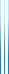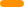# PublicationsPapers

## More complete intersection theorems

Yuval Filmus
Disc. Math. vol. 342(10), 128–142, 2019

We extend the weighted Ahlswede–Khachatrian theorem to several new settings.

First, we extend the theorem to families on infinitely many points, showing that for most settings of the parameters, no new behavior is encountered.

Second, we extend the theorem to the Hamming scheme $\mathbb{Z}_m^n$, in which a family is $t$-intersecting if any two vectors have $t$ coordinates on which the values differ by less than $s$ (this corresponds to $p=s/m$).

Third, we extend the theorem to the continuous analog of the Hamming scheme, a power of the unit circle, in which a family is $t$-intersecting if any two vectors have $t$ coordinates on which the values differ by less than $p$.

## BibTeX

@article{Filmus2019,
author = {Yuval Filmus},
title = {More complete intersection theorems},
journal = {Discrete Mathematics},
year = {2019},
month = 1,
volume = {342},
number = {1},
pages = {128--142}
}copy to clipboard